Project
Project ref
Calcs for
Date

## Beam details

 Timber strength class C24 Service class of timber 2 Timber width bt = 47 mm Timber depth ht = 120 mm Steel grade Grade 50 Steel width bs = 10 mm Steel depth hs = 100 mm Bolt diameter 12 mm## Span details

 Beam clear span Lcl = 1 m Bearing length Lb = 100 mm Beam effective span Leff = Lcl + (2 × (Lb / 2)) = 1.1 mDiagrams not to scale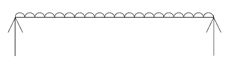Load 1: UDL - Sloping roof, 0° to 30°
 Dead load Fd,1 = 1.15 kN/m² × 8 m = 9.2 kN/m Imposed load Fi,1 = 0.75 kN/m² × 8 m = 6 kN/m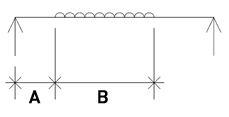Load 2: Partial UDL - Timber Dormer Wall
 Dimension to start of load, A A = 0.2 m Length of load, B B = 0.4 m Dead load Fd,2 = 1 kN/m² × 8 m = 8 kN/m Imposed load Fi,2 = 0.75 kN/m² × 8 m = 6 kN/m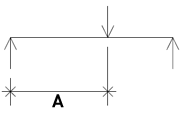## Reactions (unfactored)

 Dead Imposed Total Left reaction 7.93 kN 5.17 kN 13.10 kN Right reaction 9.53 kN 5.83 kN 15.36 kN

## Modification factors

 Timber service class modification factor K2 as table 16 Bending parallel to grain K2,ben = 1.00 Compression perpendicular to grain K2,per = 1.00 Shear parallel to grain K2,shr = 1.00 Mean & min modulus of elasticity K2,mod = 1.00 Load duration factor K3 = 1.25 From BS5268-2 Table 18, bearing is < 75mm from joist end. Bearing modification factor K4 = 1.00 Depth factor K7 = (300 / h)0.11 = 1.11 Load sharing modification factor (BS5268-2 clause 2.10.11) K8 = 1.10 Modulus of elasticity modification factor (BS5268-2 clause 2.9) K9 = 1.14

## Modular ratio of steel to timber

 Timber minimum modulus of elasticity Emin = 7,200 N/mm² Modulus of elasticity for grade 50 steel Est = 205,000 N/mm² The minimum modulus of elasticity modified by the factor K9 should be used for deflections E = Emin × K2,mod × K9 = 8,210 N/mm² Modular ratio MR = Est / E = 25

## Section properties

 Equivalent timber Area of section EA = No. steel pieces × MR × bs × ds + No. timber pieces × (bt × dt) = 36,300 mm² Inertia of timber about xx axis It = No. timber members × bt × dt³ / 12 = 13,500,000 mm4 Modified Inertia of steel about xx axis Is = No. steel plates × MR × bs × ds³ / 12 = 20,800,000 mm4 Total Inertia about xx axis in equivalent timber Ixx = It + Is = 34,300,000 mm4 Distance to edge of steel Ys = ds / 2 = 50 mm Distance to edge of timber Yt = dt / 2 = 60 mm Extreme fibre is timber section Yc = Yt = 60 mm Dist of centroid to steel edge Yn = Ys = 50 mm Z to top edge of timber Zc = Ixx / Yc = 572,000 mm³ Average density for C24 grade timber (BS 5268-2:2002 Table 8) ρmean = 420 kg/m³ Self weight of timber (g = 9.81 m/s²) Fself, timber = (bt × ht × ρmean) × Leff × g = 51.1 N Self weight of steel (g = 9.81 m/s²) Fself, steel = (bs × hs × ρsteel) × Leff × g = 84.7 N Total self weight (g = 9.81 m/s²) Fself = 136 N

## Section design parameters

 Design bending moment Mb = 4,260,000 Nmm Design shear force Fve = 15,400 N

## Check bending stress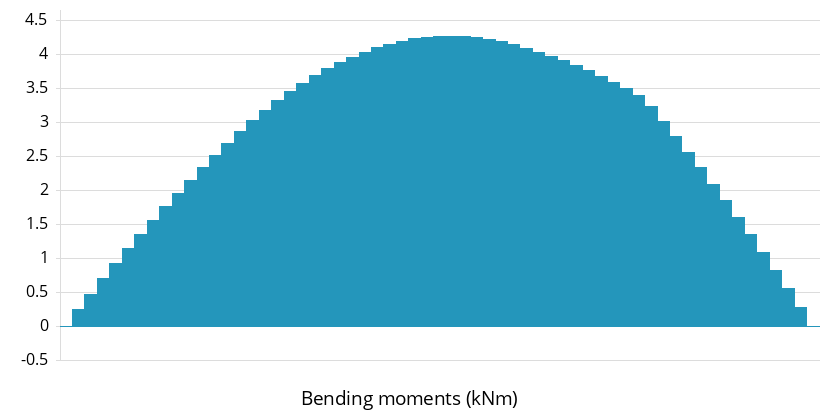Strains in the timber and steel are the same at the same distance from the neutral axis. Since Yt > Ys ( 60 mm > 50 mm ) the modified steel stress will be less than that of the timber as a ratio of the distances Ys and Yt. Timber grade bending stress parallel to grain (BS5268-2 Table 8) σt,m,g,par = 7.5 N/mm² Permissible timber bending stress (factored) σt,m,adm = σt,m,g,par × K2,ben × K3 × K7 × K8 = 11.4 N/mm² Maximum bending moment M = 4.26 kNm Applied bending stress in timber σt,m,max = M/Zc = 7.44 N/mm² Pass    σt,m,max <= σt,m,adm ( 7.444 N/mm² <= 11.406 N/mm² ) applied bending stress in timber within permissible Permissible steel stress from table 2 BS449, grade 50 steel, with a fully restrained section assumed in design, steel plate less than or equal to 40 mm thick σs,adm = 230 N/mm² Applied bending stress in steel σs,m,max = σt,m,max × MR × Yn / Yc = 155 N/mm² Pass    σs,m,max <= σs,adm ( 154.927 N/mm² <= 230 N/mm² ) applied bending stress in steel within permissible

## Check deflection (including shear deflection as required by clause 2.10.7)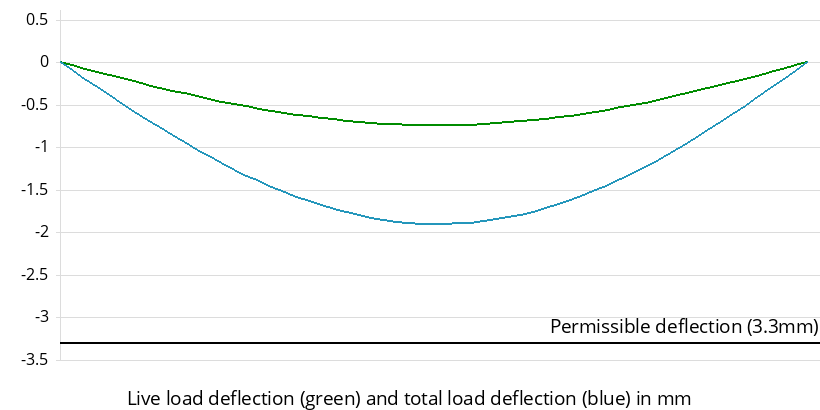## Check shear stress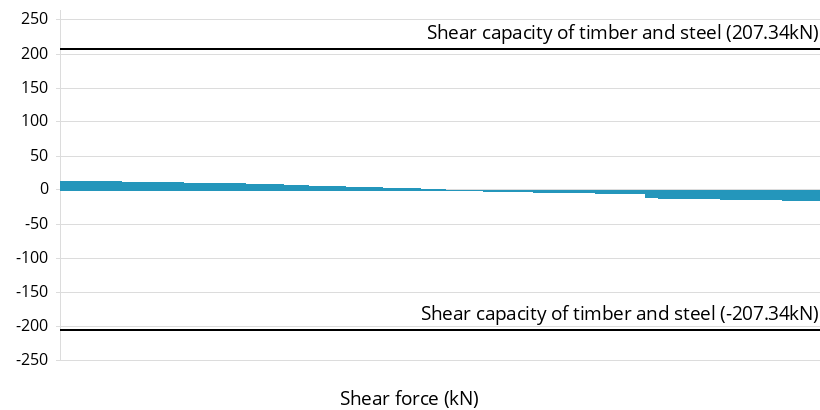## Check bearing stress

 Timber grade compressive stress perpendicular to grain (BS5268-2 Table 8) σt,c,g,⊥ = 1.9 N/mm² Permissible compressive stress perpendicular to grain (factored) σt,c,adm = σt,c,g,⊥ × K2,per × K3 × K4 × K8 = 2.61 N/mm² Timber bearing stress on support σt,c,max = Fve/(Lb × No. timber members × bt) = 1.63 N/mm² Pass    σt,c,max <= σt,c,adm ( 1.634 N/mm² <= 2.613 N/mm² ) bearing stress is less than permissible timber stress, therefore OK

## Bolts between timber and steel plates along beam

 Total load on beam W = total left reaction + total right reaction = 28.5 kN Maximum reaction at bearing Rmax = 15.4 kN Basic bolt shear capacity (tables 69-73) Vcapacity = 2.23 kN Number of interfaces Ninterfaces = 2 Minimum number of bolts required at bearings calc Nbolts, bearings, calc = Rmax / (Ninterfaces × Vcapacity) = 3.44 Limiting bolt spacing Slimit = min(2.5 × ht, 600) = 300 mm Minimum number of bolts along length of beam Nbolts_along_length = W / (Ninterfaces × Vcapacity) = 6.38 Bolts to be staggered along the length of the beam, alternately set ht/4 = 30 mm above and below the centre line. Bolts are to be spaced at 300 mm maximum centres. Bolts at the supports are to be located 0.5 x the bearing length (Lb) = 50 mm from the inner edge of the support. Minimum end and edge distances in the timber are to be 4 x bolt diameter = 48 mm. Under point loads, provide an additional bolt at the centre line of the beam.

## Design summary

 Permissible Applied/Actual Utilisation Result Shear force (kN) 207 15.4 7.4 % OK Timber bending stress (N/mm²) 11.4 7.44 65.3 % OK Steel bending stress (N/mm²) 230 155 67.4 % OK Bearing stress (N/mm²) 2.61 1.63 62.5 % OK Deflection (mm) 3.3 2.18 66.1 % OK

## Notes

This design is in accordance with BS 5268-2:2002 Structural use of timber - Part 2: Code of practice for permissible stress design, materials and workmanship, and BS449 - Specification for the use of structural steel in building.

Timber to be covered, this calculation is not to be used for timber which is fully exposed to the elements.

Wane as allowed in BS 4978:2007 + A2:2017 is permitted.

DEMO